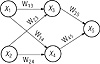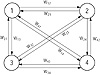# 11.C++ Neural Networks and Fuzzy Logic by Valluru B. Rao M&T Books, IDG Books Worldwide, Inc. ISBN: 1558515526   Pub Date: 06/01/95

### Example—A Feed-Forward Network

A sample feed-forward network, as shown in Figure 1.2, has five neurons arranged in three layers: two neurons (labeled x1 and x2) in layer 1, two neurons (labeled x3 and x4) in layer 2, and one neuron (labeled x5) in layer 3. There are arrows connecting the neurons together. This is the direction of information flow. A feed-forward network has information flowing forward only. Each arrow that connects neurons has a weight associated with it (like, w31 for example). You calculate the state, x, of each neuron by summing the weighted values that flow into a neuron. The state of the neuron is the output value of the neuron and remains the same until the neuron receives new information on its inputs.Figure 1.2  A feed-forward neural network with topology 2-2-1.

For example, for x3 and x5:

` x3 = w23 x2 + w13 x1 x5 = w35 x3 + w45 x4 `

We will formalize the equations in Chapter 7, which details one of the training algorithms for the feed-forward network called Backpropagation.

Note that you present information to this network at the leftmost nodes (layer 1) called the input layer. You can take information from any other layer in the network, but in most cases do so from the rightmost node(s), which make up the output layer. Weights are usually determined by a supervised training algorithm, where you present examples to the network and adjust weights appropriately to achieve a desired response. Once you have completed training, you can use the network without changing weights, and note the response for inputs that you apply. Note that a detail not yet shown is a nonlinear scaling function that limits the range of the weighted sum. This scaling function has the effect of clipping very large values in positive and negative directions for each neuron so that the cumulative summing that occurs across the network stays within reasonable bounds. Typical real number ranges for neuron inputs and outputs are –1 to +1 or 0 to +1. You will see more about this network and applications for it in Chapter 7. Now let us contrast this neural network with a completely different type of neural network, the Hopfield network, and present some simple applications for the Hopfield network.

### Example—A Hopfield Network

The neural network we present is a Hopfield network, with a single layer. We place, in this layer, four neurons, each connected to the rest, as shown in Figure 1.3. Some of the connections have a positive weight, and the rest have a negative weight. The network will be presented with two input patterns, one at a time, and it is supposed to recall them. The inputs would be binary patterns having in each component a 0 or 1. If two patterns of equal length are given and are treated as vectors, their dot product is obtained by first multiplying corresponding components together and then adding these products. Two vectors are said to be orthogonal, if their dot product is 0. The mathematics involved in computations done for neural networks include matrix multiplication, transpose of a matrix, and transpose of a vector. Also see Appendix B. The inputs (which are stable, stored patterns) to be given should be orthogonal to one another.Figure 1.3  Layout of a Hopfield network.

The two patterns we want the network to recall are A = (1, 0, 1, 0) and B = (0, 1, 0, 1), which you can verify to be orthogonal. Recall that two vectors A and B are orthogonal if their dot product is equal to zero. This is true in this case since

`      A1B1 + A2 B2 + A3B3 + A4B4 = (1x0 + 0x1 + 1x0 + 0x1) = 0 `

The following matrix W gives the weights on the connections in the network.

`       0   -3    3   -3      -3    0   -3    3 W =   3   -3    0   -3      -3    3   -3    0 `

We need a threshold function also, and we define it as follows. The threshold value [theta] is 0.

`             1  if t >= [theta] f(t) = {             0  if t < [theta] `C++ Neural Networks and Fuzzy Logic
ISBN: 1558515526
EAN: 2147483647
Year: 1995
Pages: 139

Similar book on Amazon